### Home > A2C > Chapter Ch8 > Lesson 8.2.4 > Problem8-162

8-162.
1. Find the exact value for each of the following trig expressions. For parts (g) and (h), assume that 0 ≤ θ ≤ 2π. Homework Help ✎

1.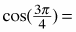2.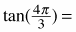3.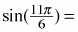4.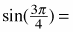5.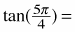6.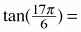7. tan(θ) = 1

8. tan(θ) = −1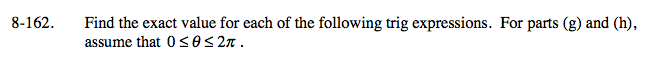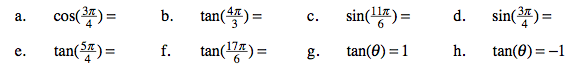Refer to problem 8-121.

$-\frac{\sqrt{2}}{2}$

$-\frac{1}{2}$

1

$\frac{\pi}{4}$